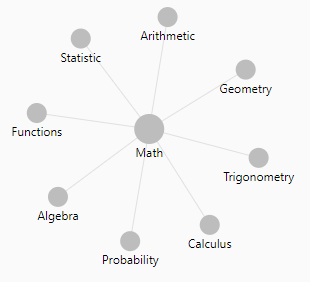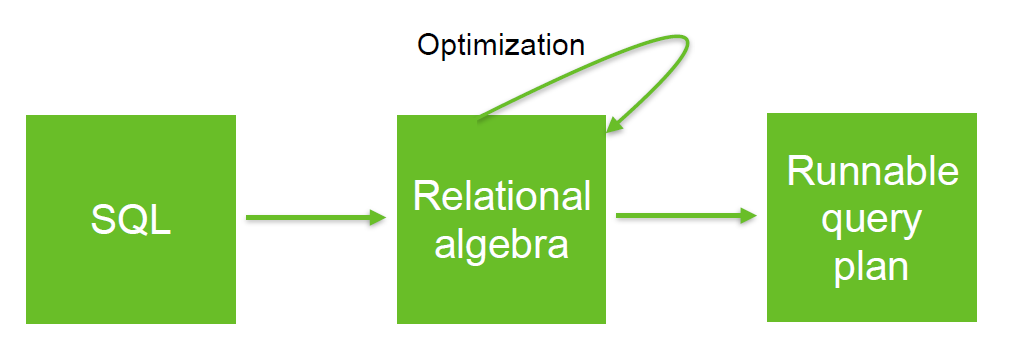# Mathematics - Algebra

A mathematical algebra has three parts:

• Objects. The collection of objects defines what we’re talking about.
• Operations
• Laws are relationships between the objects and the operations.

In high school algebra:

• the objects are numbers
• and the operations are addition, multiplication and friends.

In the algebra of Haskell types,

• the objects are types, for example Bool and Int.
• the operators take types that already exist, and generate new types from them.

Discover MoreLinear Algebra

“Linear” “algebra” is the branch of mathematics: concerning vector spaces, often finite or countably infinite dimensional, as well as linear mappings between such spaces. Such an investigation...Mathematics

Math is a language. Its algorithms are logical, its concepts proven by deductive and inductive reasoning. Math models the world. The...Relational Algebra - Expression and Operators

Relational algebra is based upon the fact that you can pass tabular data through a set of data operators (select, filter, join, sort, union, etc.) in a algebraic structure. It means that: the output...